# fx-991EX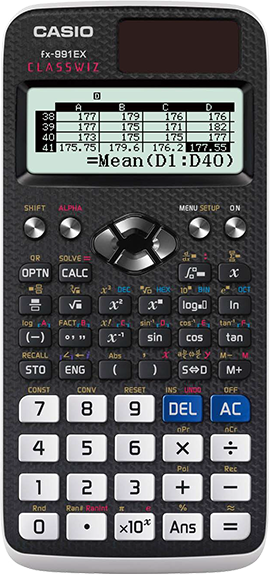# fx-991EX

## The New Classroom Standard With High-resolution LCD Technology

Ideal non-programmable scientific calculator for technology integrated teaching and learning based on the Philippines Ｋ-12 curriculum for Lower / Upper Secondary schools and University.
fx-991EX can assist teaching and learning of SHS core subjects as well as SHS academic tracks, especially STEM strand, ABM strand and HUMMS strand.

• ### Presentation

Introduces the excellent features of the new ClassWiz

• ### Function

Introduces high-performance functions effective for advanced mathematics

# Features

## 1 Easy and Intuitive operability

Intuitive and interactive operation makes it easy to use the features of ClassWiz.
By the use of icons, selection of the desired function is quick and easy.

Visual, interactive and textbook-based displays make operation easier for teaching the use of scientific calculators, so you can concentrate on teaching mathematics.

•ClassWiz Series

•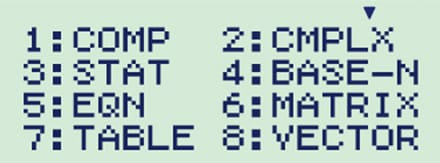ES PLUS Series

## Interactive format

Interactive menu display realizes more intuitive operation.

••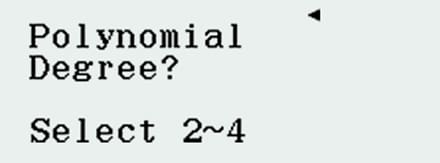•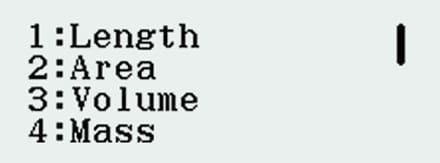•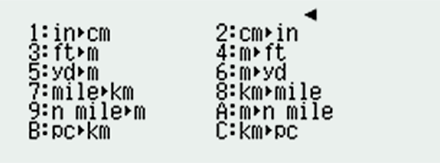## Natural Textbook Display Models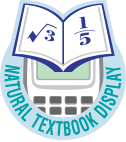Input and display fractions, powers, logarithms, roots, and other mathematical formulas and symbols just as they appear in textbooks.

•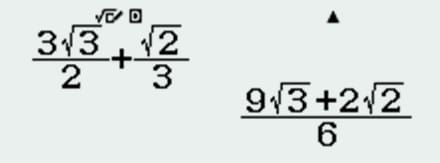ClassWiz Series

•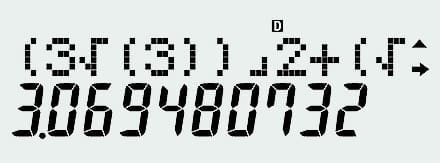MS Series

## Example

• Solve the simultaneous equations.
{ x + 2y = 3 2x +3y = 4

• The solution is
x = -1
y = 2

### ClassWiz Series

•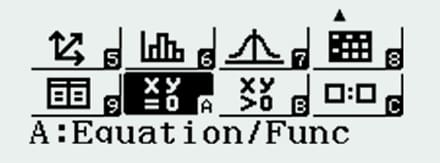Icon display

•Select equation

•Input coefficient

•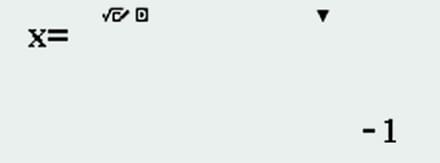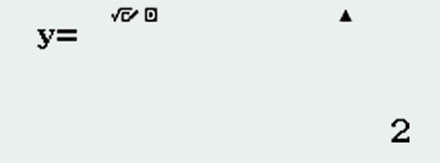Solution

### MS Series

•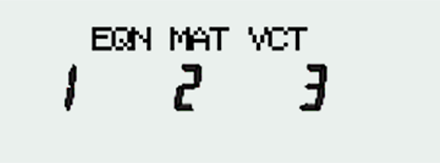Icon display

•Select equation

•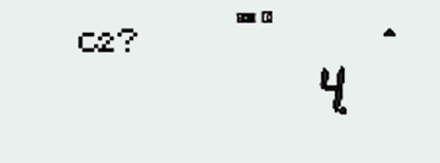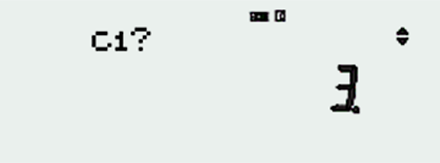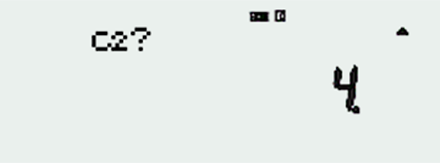Input coefficient

•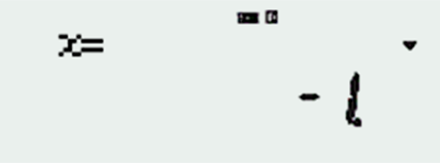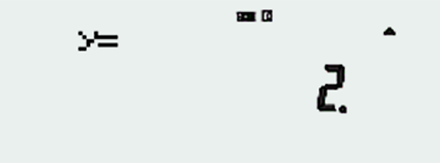Solution

## 2 Essential functions for learning all in one

A wide range of essential functions are effective for education and available to support student's learning.

•Useful for learning statistics.
Up to 5 columns x 45 rows
(maximum of 170 data items)

## 1Essential functions

Even advanced mathematical calculations are simple to perform and functions that take advantage of previous unavailable display capabilities are now possible.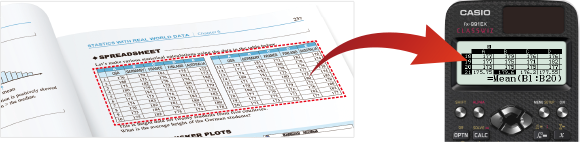#### Statistics

The spreadsheet function can be used for studying statistics which has become important in mathematics classes.

### Example: Student's height data comparison chart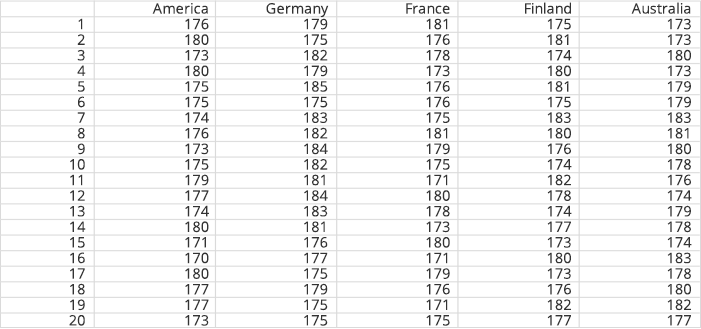### Statistical calculation such as the mean of the data is executed

•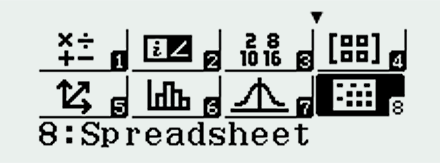•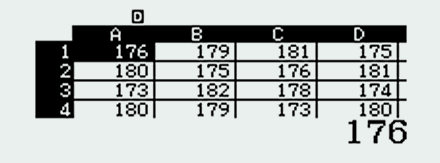•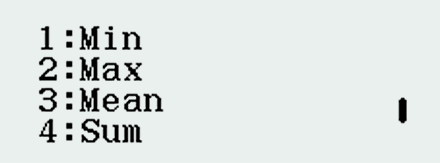•#### Recursion

In addition, the spreadsheet function makes it possible to enter recursive formulas.

Sequence: a1=2 and an+1 -2an-3 (n=1, 2, 3 ...)

•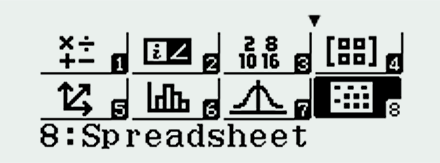•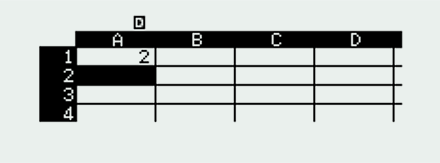••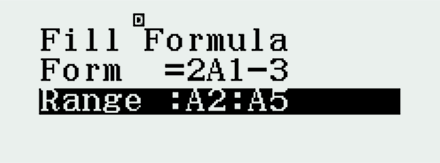•a1-2, a2=1, a3=-1, a4=-5, a5=-13

#### Riemann Integral

Calculation using advanced Riemann functions is also possible.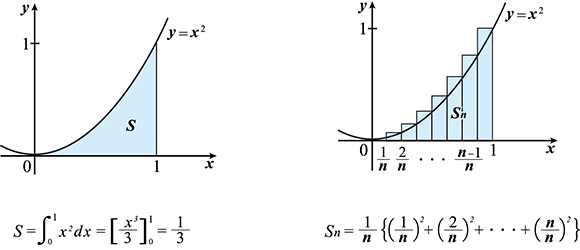•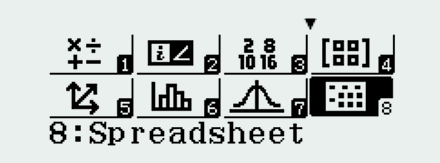•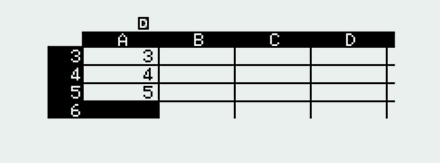•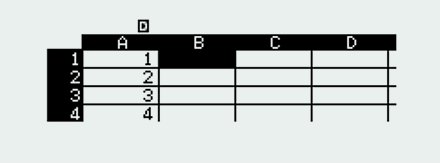••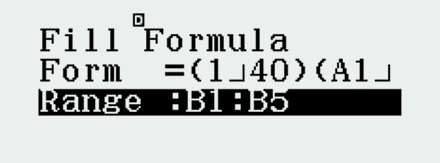•The spreadsheet function makes it easy to use real-world data even in the classroom, enabling students to effectively study statistical processing.

## 2Variety of functions available to support student's learning.

### From basic level to advanced math level for secondary and tertiary education.

•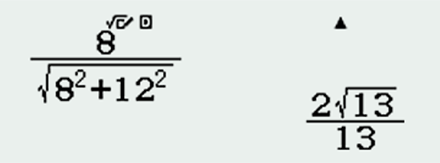Fractions and rational numbers

•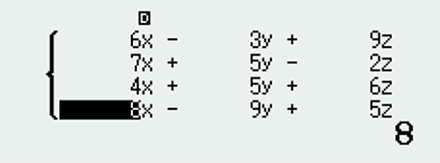Equation

•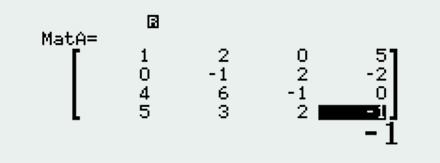Matrix

•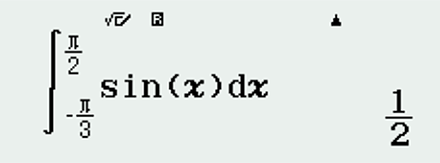Integration

## Example

Solve the matrix calculation.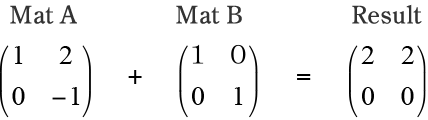### ClassWiz Series

•Able to enter Matrix just as textbook form

•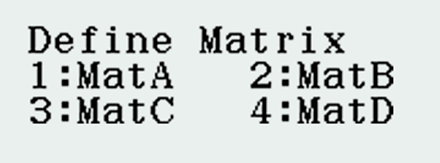••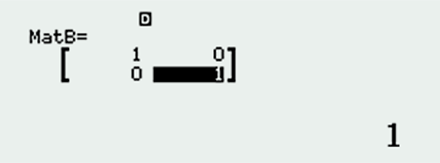•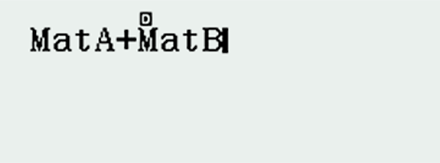•Able to have the answer in 1 display as textbook form

### MS series

•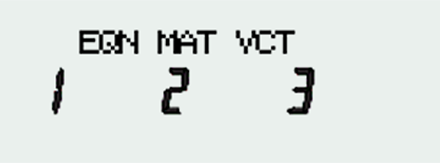Need 4 displays to enter numbers in Matrix A

•Need 4 displays to enter numbers in Matrix B

•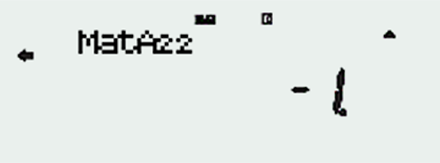••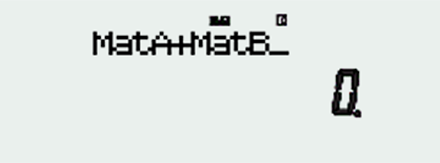•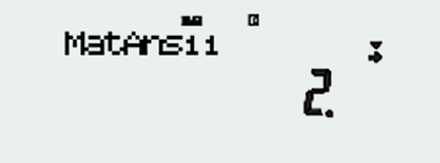Need 4 displays to check the final answer

## 3 Simple and quick calculations

Simple key strokes and visualized formulas make calculations faster.
Fast calculation for smooth performance in class or during examinations.

•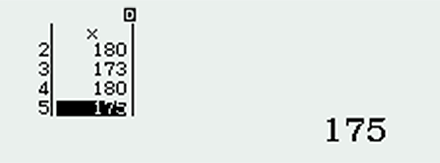•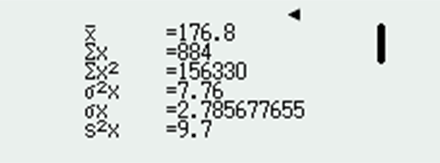## Example

Calculate the mean height of 5 students.

< Students’ height >
176cm, 180cm, 173cm, 180cm, 175cm

### ClassWiz series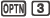2 steps

•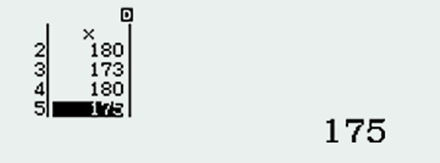•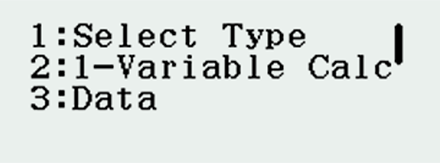•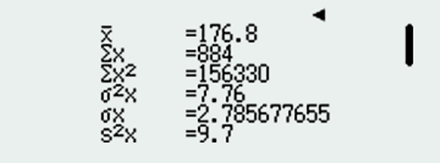Fewer keystrokes and faster !!
Standard variation, variance, mean, sum all in one display!
No more jotting down each solution on paper, ClassWiz will save your time.

### ES PLUS Series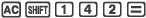6 steps

•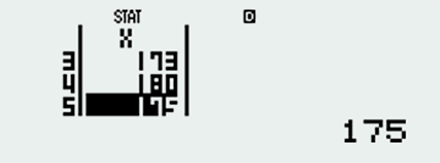•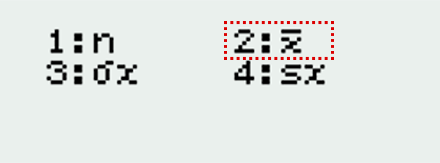•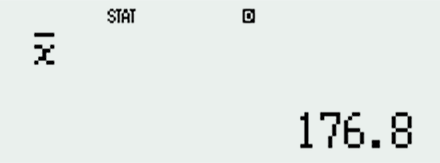### ES PLUS Series6 steps

•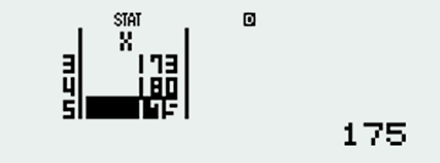•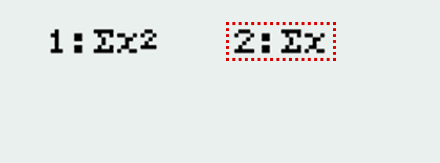•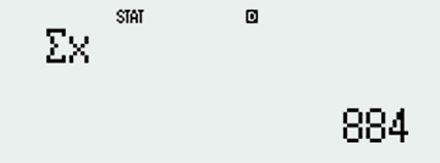### ES PLUS Series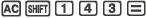6 steps

••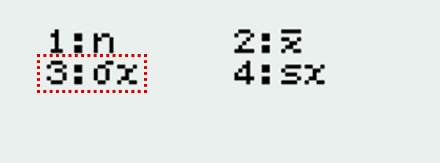•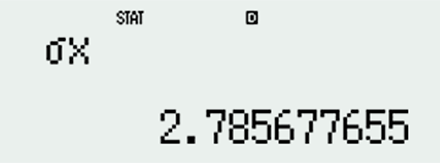## 4 Extensive and advanced service using QR Code

Generate QR Codes of equations input into the calculator by a simple operation.
Graphs and other information can be displayed on smartphone or tablet screens.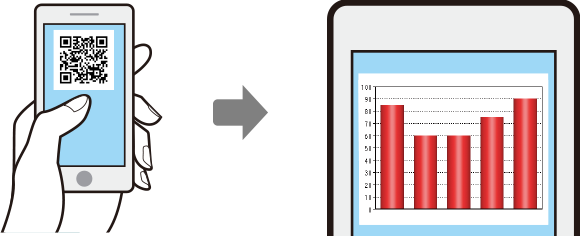## 1Visualization with graphs

• STEP 1
Input formula
•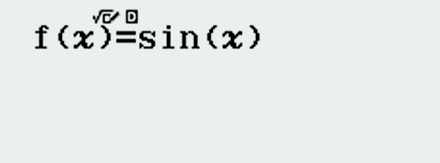• STEP 2
Table
•• STEP 3
QR Code
•• STEP 4
Display Graph
••Makes formulas visually understandable

## 2Online Sharing

Online sharing system assists active learning in your classroom.
If you use the QR Code Function, you can share students' results.
It will be a great help for group-work lessons.

### Group A

•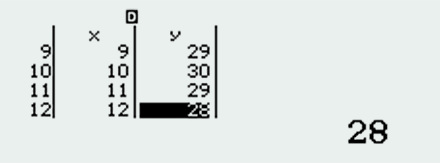Input data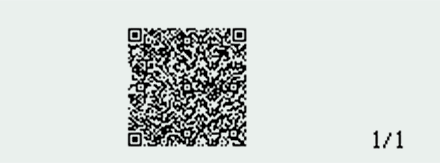QR Code

•Graph

### Group B

•Input dataQR Code

•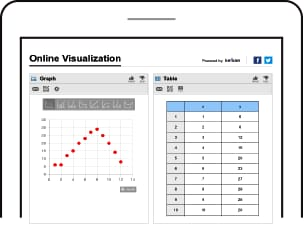Graph

• ### Group discussion

Combine and compare graphs on a single screen.

•Combine Group A, Group B

## 3Quick user manual call-up

• STEP 1
•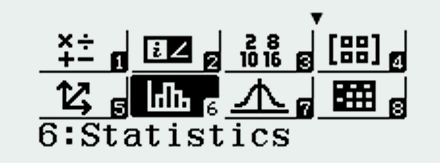• STEP 2
QR Code
•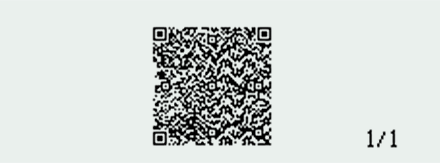• STEP 3
Display the manual
••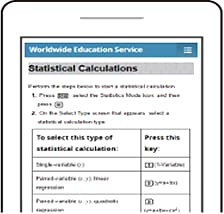Makes learning to operate the calculator fast and easy

### Please see the website in detail

QR code portal site
http://wes.casio.com# Teacher's Voice## School

Math and Science Division Chair at Lyons Township High School
LaGrange Illinois (USA)

Ismael Zamora

## Comment

I love the simplicity of the Casio fx-991EX (ClassWiz). The use of a natural textbook display and high resolution screen allows me to present mathematics the way students see mathematics in their textbooks. The ClassWiz also allows me to teach concepts I never could before. Statistics, data analysis, probability and spreadsheets are all now within the realm of possibility. My favorite feature is the QR Code generator. I now have the power of a graphing calculator in the hands of every student. The ClassWiz allows me to focus on the learning and not the button pushing.